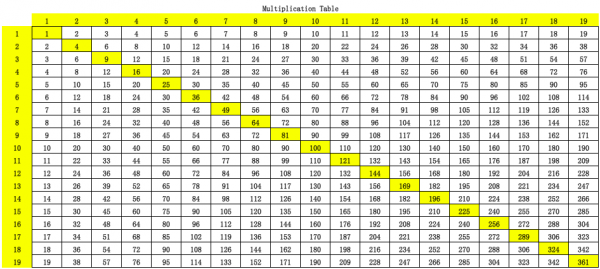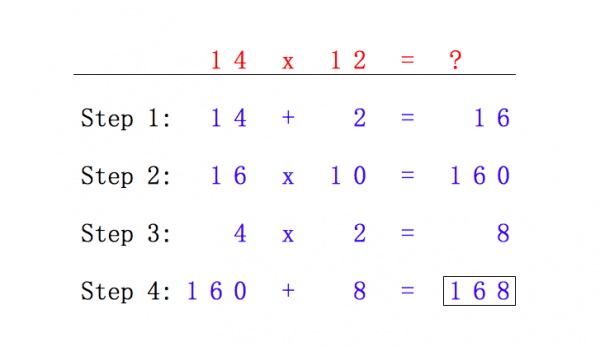# Indian Multiplication Table

## >> More DIY Ideas <<While our kids at school are struggling with the common core standards, their peers at the other end of the earth can memorize the entire 19×19 times table! Only looking at this Indian multiplication table makes my head spin.

My Indian friends give me a little trick to memorize this table. I suddenly got it after mastered this magical trick. I feel obligated to share with my readers. That’s why India is developing so fast lately!

Master this trick. Hope we don’t struggle as much in math. Here is how to memorize 19×19 multiplication table.

First try to calculate in your mind:

14 x 12 = ?

Struggling, right? The following is how Indian does it.Step 1: First add the second digit of the second number into the first number.

14 + 2 = 16

Step 2: Multiply the first answer by 10, which is merely add a zero to its end.

16 x 10 = 160

Step 3: Multiply the last digit of the two numbers. In this example

4 x 2 = 8

Step 4: Add the answers from step 2 and step 3 to get the answer of the original question.

160 + 8 = 168

So the answer is 168. Isn’t it simple? Here are a couple more example.

17 x 16

1. 17 + 6 = 23
2. 23 x 10 = 230
3. 7 x 6 = 42
4. 230 + 42 = 272

19 x 19

1. 19 + 9 = 28
2. 28 x 10 = 280
3. 9 x 9 = 81
4. 28 + 81 = 361

Now can you try?

13 x 18 = ?

If you still can’t memorize them, you may want to try this math trick by doing multiplication with fingers.

## Get New Posts Right To Your Inbox!

Get captivating new Tutorials, just like this one, delivered right to your inbox each day. Just sign up and we will send you the best new tutorials as they come in. Your email address will NEVER been spammed, sold, or shared. You are welcome to unsubscribe at any time with the link in the email.